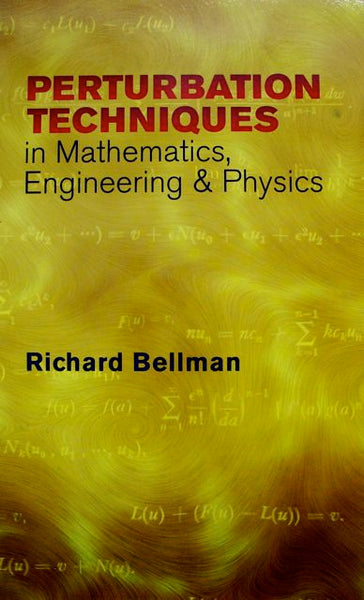# Perturbation Techniques in Mathematics, Engineering& Physics

: In stock

: Dover Publications

: 1110000003531

: Book

\$9.95

#### Details

The perturbation techniques begins by solving linear algebraic equation that exhibits versatility of the Lagrange expansion theorem. The second part concentrates on nonlinear differential equations, presenting renormalization techniques. By concluding the chapters variety of questions centering on a second-order linear differential equation.

• Isbn-10 Digit: 0486432580
• Author-1: Richard Bellman